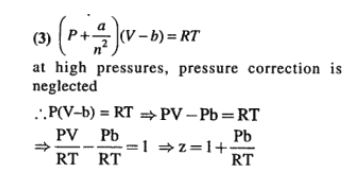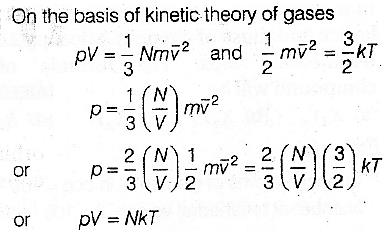Courses

# Retro (Past 13 Years) JEE Main (States Of Matter)

## 13 Questions MCQ Test Chemistry for JEE Advanced | Retro (Past 13 Years) JEE Main (States Of Matter)

Description
This mock test of Retro (Past 13 Years) JEE Main (States Of Matter) for JEE helps you for every JEE entrance exam. This contains 13 Multiple Choice Questions for JEE Retro (Past 13 Years) JEE Main (States Of Matter) (mcq) to study with solutions a complete question bank. The solved questions answers in this Retro (Past 13 Years) JEE Main (States Of Matter) quiz give you a good mix of easy questions and tough questions. JEE students definitely take this Retro (Past 13 Years) JEE Main (States Of Matter) exercise for a better result in the exam. You can find other Retro (Past 13 Years) JEE Main (States Of Matter) extra questions, long questions & short questions for JEE on EduRev as well by searching above.
QUESTION: 1

Solution:
QUESTION: 2

Solution:
QUESTION: 3

### The compressibility factor for a real gas at high pressure is (AIEEE 2012)

Solution:QUESTION: 4

a and b are van der Waals’ constants for gases. Chlorine is more easily liquefied than ethane because

(AIEEE 2011)

Solution:
QUESTION: 5

The molecular velocity of any gas is

(AIEEE 2011)

Solution: Acc to kinetic theory of gases 1/2MU² is proportional to T. Therefore U is proportional to √T. Where U is average velocity of the gaseous molecules
QUESTION: 6

When r, p and M represent rate of diffusion, pressure and molecular mass, respectively, then the ratio of the rates of diffusion (rA / rB) of two gases A and B, is given as

(AIEEE 2011)

Solution:
QUESTION: 7

If 10-4 dm3 of water is introduced into a 1,0 dm3 flask at 300 K, then how many moles of water are in the vapour phase when equilibrium is established?

(Given, vapour pressure of H2O at 300 K is 3170 Pa; R = 8.314 JK-1 mol-1)

(AIEEE 2010)

Solution:
QUESTION: 8

Which one of the following statements is not true about the effect of an increase in temperature on the distribution of molecular speeds in a gas?

(AIEEE 2005)

Solution:
QUESTION: 9

As the temperature is raised from 20°C to 40°C, the average kinetic energy of neon atoms changes by a factor of which of the following?

(AIEEE 2004)

Solution:
QUESTION: 10

In van der Waals' equation of state of the gas law, the constant 'b' is a measure of

(AIEEE 2004)

Solution:
QUESTION: 11

According to the kinetic theory of gases, in an ideal gas, between two successive collisions a gas molecule travels

(AIEEE 2003)

Solution: They move in zig-zag motion.
QUESTION: 12

Based on kinetic theory of gases following laws can be proved

(AIEEE 2002)

Solution:QUESTION: 13

For an ideal gas, number of moles per litre in terms of its pressure p, temperature T and gas constant R is

(AIEEE 2002)

Solution: